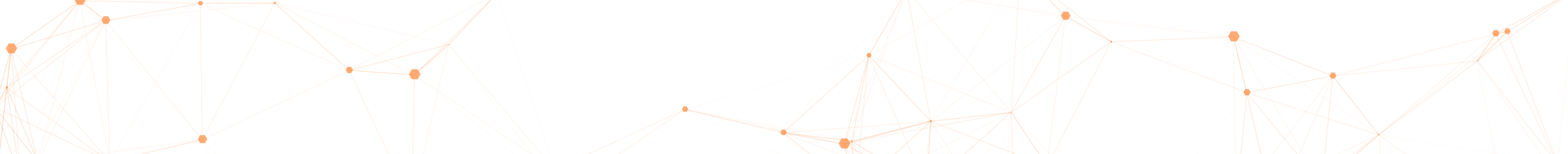# IMBM, İstanbul, Turkey## Speakers

• Hakan Ayral (Galatasaray University)
• Merve Durmus (Galatasaray University, Yeditepe University)
• Muhammed Uludag (Galatasaray University )
• Ayberk Zeytin (Galatasaray University )

## Program - 16.02.2013

10.30 - 10.50 Merve Durmus

11.15 - 12.15 Muhammed Uludag

13.45 - 14.45 Ayberk Zeytin

15.00 - 16.00 Hakan Ayral

## Abstracts

• Hakan Ayral (Galatasaray University)
• Title : Automated Theorem Proving Systems

Abstract: Automated theorem proving is formal proving of mathematical theorems by computer programs with little or no user intervention.
A formal proof is a proof in which every single logical inference has been checked back to the fundamental axioms of mathematics; first serious attempt to formalize mathematics in terms of symbolic logical inferences was by Russel and Whitehead in their Principia Mathematica, written with a purpose to derive some of the mathematical expressions of number theory.
In a consistent axiomatic system, the validity of a theorem can be expressed as the satisfiability of a logic formula which reduces to a set of propositional satisfiability problems. The problem of deciding the satisfiability of a formula (SAT-problem) varies from trivial to impossible; for the case of propositional logic, the problem is decidable but Co-NP-complete.
First-order theorem proving is one of the most mature subfields of automated theorem proving as first order logic is expressive enough to allow the specification of arbitrary problems, and a number of sound and complete calculi have been developed, enabling fully automated systems.
As part of the talk we will present some of the widely known automated theorem proving softwares and browse through formalizations of some trivial facts (2+2=4, primality of 3) and some non-trivial proofs.

• Merve Durmus (Galatasaray University, Yeditepe University)
• Title: Subgroups of the infinite dihedral group and graphs

Abstract: In this talk we will show how one constructs a unique graph to every subgroup of the infinite dihedral group, which may be thought of as the toy case of the modular group to be described in the next talk.

• Muhammed Uludag (Galatasaray University )
• Title: Binary quadratic forms as dessins

Abstract: Since it encodes the Euclidean algorithm, the modular group is a very fundamental object in mathematics. It acts by conjugation on an infinite bipartite planar tree, which we call the Farey tree. The orbits are graphs with one cycle which we call çarks. We show that each çark represents naturally the class of an indefinite binary quadratic form, and every such class can be represented this way. Given a çark, if we specify an edge together with an orientation of its cycle, then this determines an indefinite binary quadratic form belonging to the class represented by the çark, and every such form can be represented this way. This work is funded by TUBITAK110T690 and GSU12.504.001 grants.
(Joint work with M. Durmuş and A. Zeytin)

• Ayberk Zeytin (Galatasaray University )
• Title: Thompson's Groups and the Modular Group

Abstract: In this talk after defining Thompson's groups F and T via universal Teichmuller theory, we will describe a new point of view on the Thompson's groups (F and T) using the modular group. Then we will discuss the category of carks, a category whose objects are indefinite binary quadratic forms and morphisms are flips.
(this is joint work with M.Uludag.)

## Yer

IMBM: Istanbul Matematiksel Bilimler Merkezi

© GSÜ Math. Tüm hakları saklıdır.